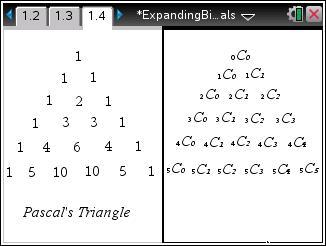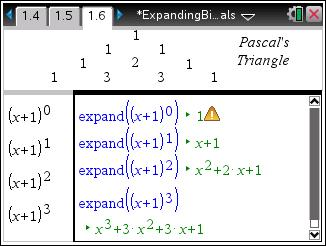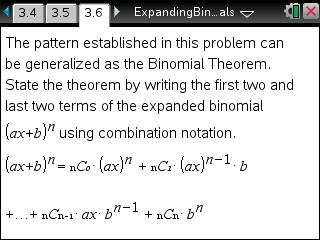# Activities

••• ##### Subject Area

• Math: Precalculus: Sequences
• Math: Precalculus: Series

• ##### Author9-12

45 Minutes

• ##### Device
•TI-Nspire™ CX/CX II
•TI-Nspire™ CX CAS/CX II CAS
• TI-Nspire™ Navigator™
• TI-Nspire™
• TI-Nspire™ CAS
• ##### Software

TI-Nspire™
TI-Nspire™ CAS

3.2

## Expanding Binomials

#### Activity Overview

In this activity, students will explore the link between Pascal's Triangle and the expansion of binomials in the development of the Binomial Theorem.

#### Key Steps

•In this activity, students will explore Pascal’s Triangle and the Binomial Theorem. They will compare the numbers in the triangle to combinations.

•First, they will expand binomials to investigate the effect of b in the binomial (x + b)n and then see the effect of a in the binomial (ax + 1)n.

•They will then develop a pattern for both variables together in the binomial (ax + b)n in order to state the Binomial Theorem. There are extra problems on the worksheet for students to complete using the theorem.